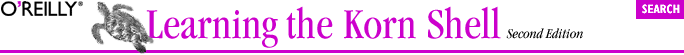home | O'Reilly's CD bookshelfs | FreeBSD | Linux | Cisco | Cisco Exam6.2. Numeric Variables and Arithmetic

We can use +%j to print a little holiday anticipation message:

print "Only \$(( (365-\$(date +%j)) / 7 )) weeks until the New Year!"

While expr and ksh88 were limited to integer arithmetic, ksh93 supports floating-point arithmetic. As we'll see shortly, you can do just about any calculation in the Korn shell that you could do in C or most other programming languages.

Korn shell arithmetic operators are equivalent to their counterparts in the C language. Precedence and associativity are the same as in C. (More details on the Korn shell's compatibility with the C language may be found in Appendix B; said details are of interest mostly to people already familiar with C.) Table 6-2 shows the arithmetic operators that are supported, in order from highest precedence to lowest. Although some of these are (or contain) special characters, there is no need to backslash-escape them, because they are within the \$((...)) syntax.

Table 6-2. Arithmetic operators

Operator Meaning Associativity
++ -- Increment and decrement, prefix and postfix Left to right
+ - ! ~ Unary plus and minus; logical and bitwise negation Right to left
**

Exponentiation

Right to left
* / % Multiplication, division, and remainder Left to right
+ - Addition and subtraction Left to right
<< >> Bit-shift left and right Left to right
< <= > >= Comparisons Left to right
== != Equal and not equal Left to right
& Bitwise and Left to right
^ Bitwise exclusive-or Left to right
| Bitwise or Left to right
&& Logical and (short circuit) Left to right
|| Logical or (short circuit) Left to right
?: Conditional expression Right to left

= += -= *= /= %= &= ^= <<= >>=

Assignment operators Right to left
, Sequential evaluation Left to right

 ksh93m and newer. The ** operator is not in the C language.

For example, \$((3 > 2)) has the value 1; \$(( (3 > 2) || (4 <= 1) )) also has the value 1, since at least one of the two subexpressions is true.

If you're familiar with C, C++ or Java, the operators listed in Table 6-2 will be familiar. But if you're not, some of them warrant a little explanation.

The assignment forms of the regular operators are a convenient shorthand for the more conventional way of updating a variable. For example, in Pascal or Fortran you might write x = x + 2 to add 2 to x. The += lets you do that more compactly: \$((x += 2)) adds 2 to x and stores the result back in x. (Compare this to the recent addition of the += operator to ksh93 for string concatenation.)

Since adding and subtracting 1 are such frequent operations, the ++ and -- operators provide an even more abbreviated way to do them. As you might guess, ++ adds 1, while -- subtracts 1. These are unary operators. Let's take a quick look at how they work.

\$ i=5
\$ print \$((i++)) \$i
5 6
\$ print \$((++i)) \$i
7 7

What's going on here? In both cases, the value of i is increased by one. But the value returned by the operator depends upon its placement relative to the variable being operated upon. A postfix operator (one that occurs after the variable) returns the variable's old value as the result of the expression and then increments the variable. By contrast, a prefix operator, which comes in front of the variable, increments the variable first and then returns the new value. The -- operator works the same as ++, but it decrements the variable by one, instead of incrementing it.

The shell also supports base N numbers, where N can be up to 64. The notation B#N means "N base B." Of course, if you omit the B#, the base defaults to 10. The digits are 0-9, a-z (10-35), A-Z (36-61), @ (62), and _ (63). (When the base is less than or equal to 36, you may use mixed case letters.) For example:

\$ print the ksh number 43#G is \$((43#G))
the ksh number 43#G is 42

Interestingly enough, you can use shell variables to contain subexpressions, and the shell substitutes the value of the variable when doing arithmetic. For example: